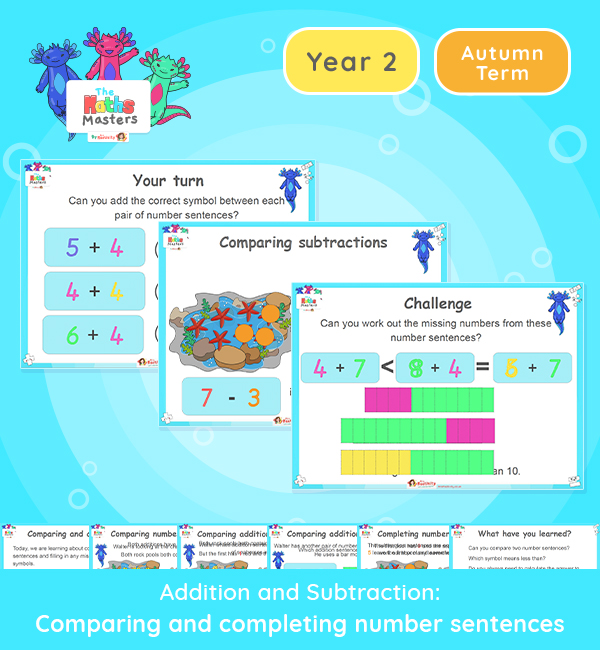# Year 2 | Comparing Number Sentences Lesson PresentationAligned with the maths mastery approach, this Year 2 | Comparing Number Sentences Lesson Presentation is fully editable, and is designed for the Year 2 maths curriculum covering the following maths objectives for the autumn term:

This lesson focuses on comparing addition/subtraction sentences and filling in the missing numbers to make number sentences equal.

Small Steps: Compare number sentences.

NC Links: • Add and subtract numbers using concrete objects, pictorial representations and mentally. • Show that the addition of two numbers can be done in any order (commutative) and subtraction of one number from another cannot. • Recognise and use the inverse relationship between addition and subtractions and use this to check calculations and solve missing number problems.

Previous Experience: Add and subtract within 10.

Future Applications: Add and subtract using mental and formal written methods.

TAF Statements: Working Towards: Add and subtract (one digit numbers) explaining their method verbally, in pictures or using apparatus.

Working At: Recall all the number bonds to and within 10, and use these to reason with and calculate bonds to and within 20, recognising other associated additive relationships.

Greater Depth: Use reasoning about numbers and relationships to solve more complex problems and explain their thinking.

Aligned with the order of teaching of the White Rose Maths scheme of work, use this to help your class get to grips with each mathematical concept. This lesson presentation also includes varied fluency activities, problem solving, and mathematical discussion questions too.

Explore our other  year 2 addition and subtraction resources.

## Recently Viewed